# INNER JOIN a Number Of Tables

Published on
16,477 Points
4,377 Views
6 Endorsements
Awarded
Community Pick
INTRODUCTION

Joining tables is a way to tell the DBMS (Access in this article) what data to hold in memory from a group of tables. The purpose of a DBMS is to store, retrieve, and analyze data. Join is a way to limit the retrieved data that is stored in memory for further processing.  Joins include cross join, inner join and outer join types.

This article addresses the inner join and introduces a way to help design the FROM clause of a SQL statement using INNER JOIN of many tables. It removes the confusion of ordering and bracketing pairs of tables in the join sequence. This is treated with reference to the way a relation is drawn in query design mode.

This is a step by step tutorial using the Access environment.  A sample database is included to reduce the effort of recreating the necessary setup needed to continue with the tutorial.

A new approach will be presented to compile the join part by knowing the order of relations between tables, and the start and end fields of each relation

AUDIENCE

You understand the need for joins between tables.
You know the types of joins between tables.
If you experience some confusion in writing  the SQL by INNER JOINing the five tables, keep reading.
If you are looking for a concrete way in writing the join clause, keep reading.

PREREQUISITES

It is assumed that you:
understand  the difference between a Table and a query;
know how to use the query designer to relate tables;
understand how to set criteria in a query grid.

OBJECTIVES

For a database of 5 tables, A(a, r, x), B(a, b, r, x), C(b, c, r, x), D(c, d, r, x), and E(d, e, r, x), and a table of relations between these tables, the reader will be able to:

write the JOIN part of the SQL statement involving required tables
rewrite the JOIN part of the SQL statement when adding, removing, or changing direction of, a relation between tables.

NOTATIONS

In this article we will introduce few notations. These notations are not intended to be added to your store of notations, but to help in a concrete understanding within this article.  We introduce the expression XYZ, a three character name, with the following simple rules.
X can be any of the following,  group1, characters:  A, B, C, D, or E
Y can be any of the following, group2, character, a….z
? is a wild card that replaces any , group2, character, a….z
Z can take any of , group3, characters,  F, or T
The following are acceptable for the XYZ notation.
» A?F. Example AaF, AxF
» B?T. Example BaT, BxT
» Two notations linked graphically are shown as follows:

»» A?F   B?T. Example AaF BaT, a relation is drawn from A.a to B.a
»» B?F   A?T. Example BaF AaF, a relation is drawn from B.a to A.a

» A?F   B?T, link is drawn from left to right.
» A?T   E?F, link is drawn from right to left

Table of relations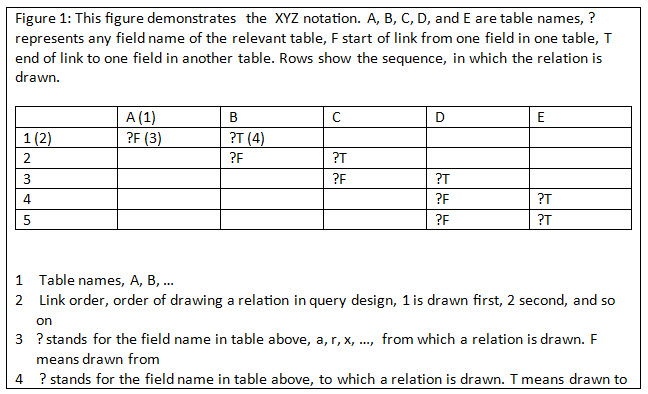The relations between the tables, as read from Figure 1 are as follows:
Single relation, and contributes to a simple criteria condition.
A?F  B?T, criteria A.? = B.?. Example. A.a =  B.a
B?F  C?T, criteria B.? = C.? . Example. B.a = A.a
C?F  D?T, criteria C.? = D.?. Example. C.a = D.a
Double relation, and contributes to a compound criteria condition.
D?F  E?T, and D?F  E?T, criteria (D.? = E.?) AND (D.? = E.?) Example. (D.a =E.a) AND (D.x = E.x)

DATABASE  SETUP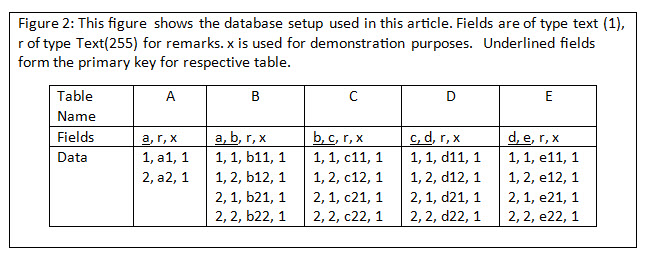A copy of the sample database is included, and includes the queries used in this article. See attached documents.
join01.accdb   join01.mdb

How to: INNER JOIN

Step 1: Open the database, and make sure you see the 5 tables. A, B, C, D, and E. as shown here.  Figure 3.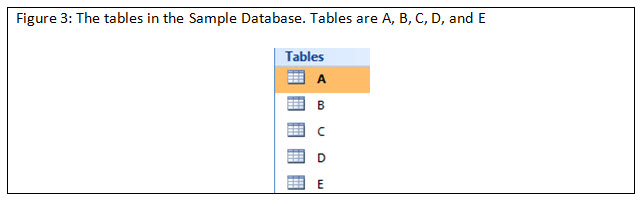Step 2: List tables, A and B, as shown bellow. Figure 4.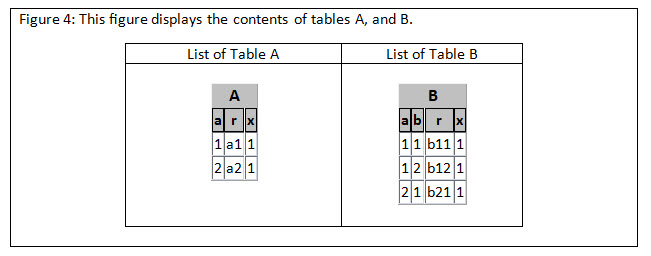Step 3: Design a query, which lists the content of tables A and B and show the design view, SQL view, and the result of running the query. Figure 5.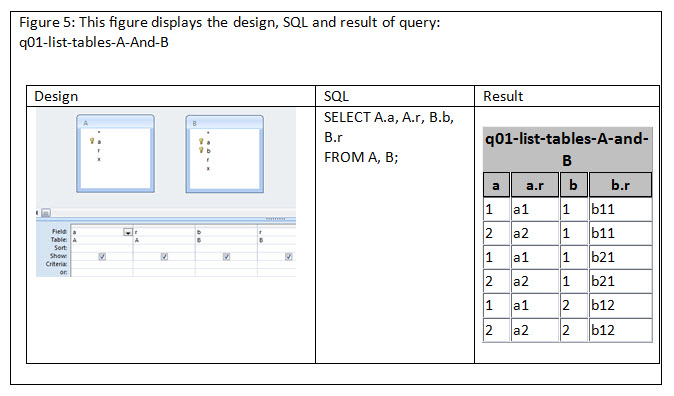This step produces the Cartesian product of tables A and B, where every record in table A is combined with every record in Table B. It is referred to as a cross join. The result shows 6 records, 2 records of A each combined with every row (3 times) in table B. The eligible records are records 1, 5, and 4, as they appear in Table 4. This is the way access pulls the records when the tables are used as such. The cross join result forms one entity stored in memory, where we can select the proper data using a criteria, or automatically by properly joining the tables, as will be seen next.

Step 4: Design a query to show the relevant records from tables A and B.  This is the same as the previous query, Step 3, but we add a criteria A.a = B.a. Figure 6.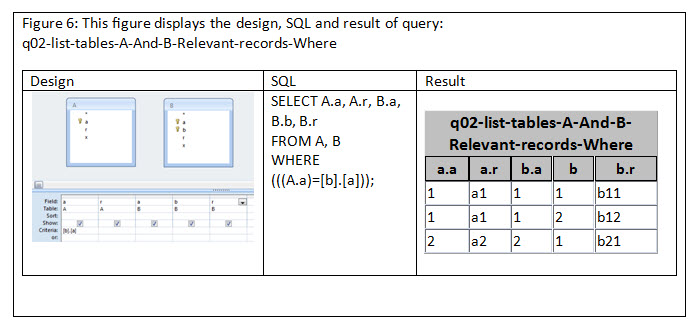Step 5: Design a query to show the relevant records from tables A and B by INNER JOINing the two to each other. The same result will be as step 4. Inner Join is a way to link two tables relating two fields that set the criteria for selecting records. Figure 7.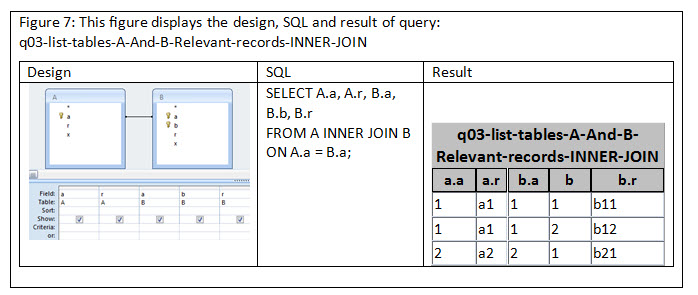Step 6: Writing INNER JOIN SQL from relations table. Figure 8.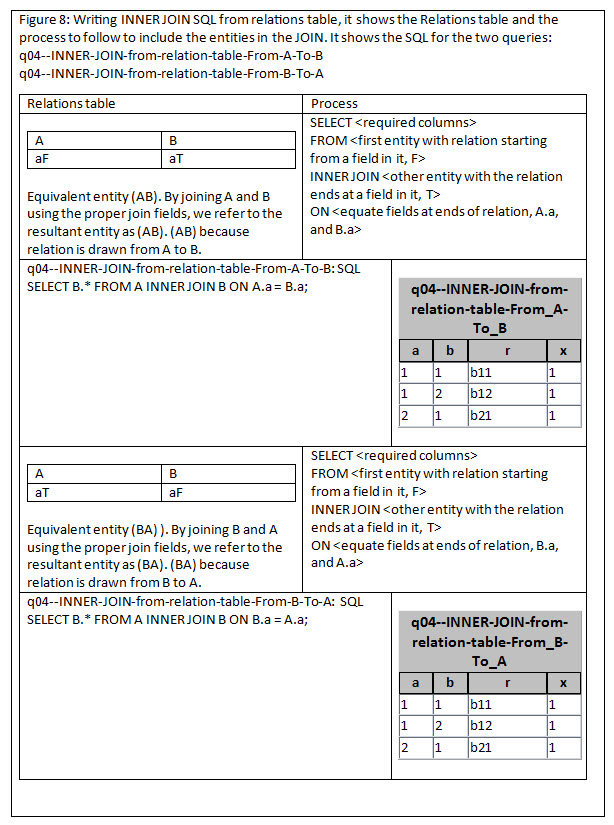The result of both queries will be the same, so you can assume any direction for the links and construct the query as shown. But be aware of that behavior when constructing the queries.
A INNER JOIN B is treated as one entity in memory, (AB) for short, this entity can be cascaded using INNER JOIN with a third table and so on. A bracket surrounds every two INNER JOINed entities. Hence nested brackets are used to join many tables.  Adding a third table C to the joined tables will result in a new entity C INNER JOIN (A INNER JOIN B), C(AB),  if the link end on C table is the starting point of the link, or (A INNER JOIN B) INNER JOIN C, (AB)C,  if the link end on the C table is the ending point. The result will be the same, but sometimes you cannot follow an ordered way of drawing links, you need to add, or delete tables and links in any order.

Steps 7, 8: Writing INNER JOIN SQL from relations table for 3 tables (entities). Figure 9.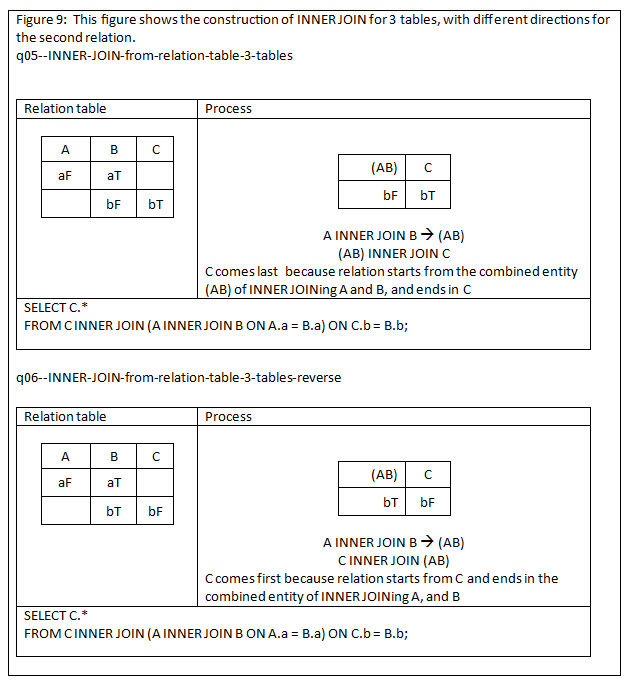Reversing the second relation, involving table C has the effect of moving C to start of entities, followed by the compound entity formed from tables A and B, which will be shown inside a bracket to represent a single resultant entity.

Step 9: Writing INNER JOIN SQL from relations table for 5 tables (entities. General method). Figure10.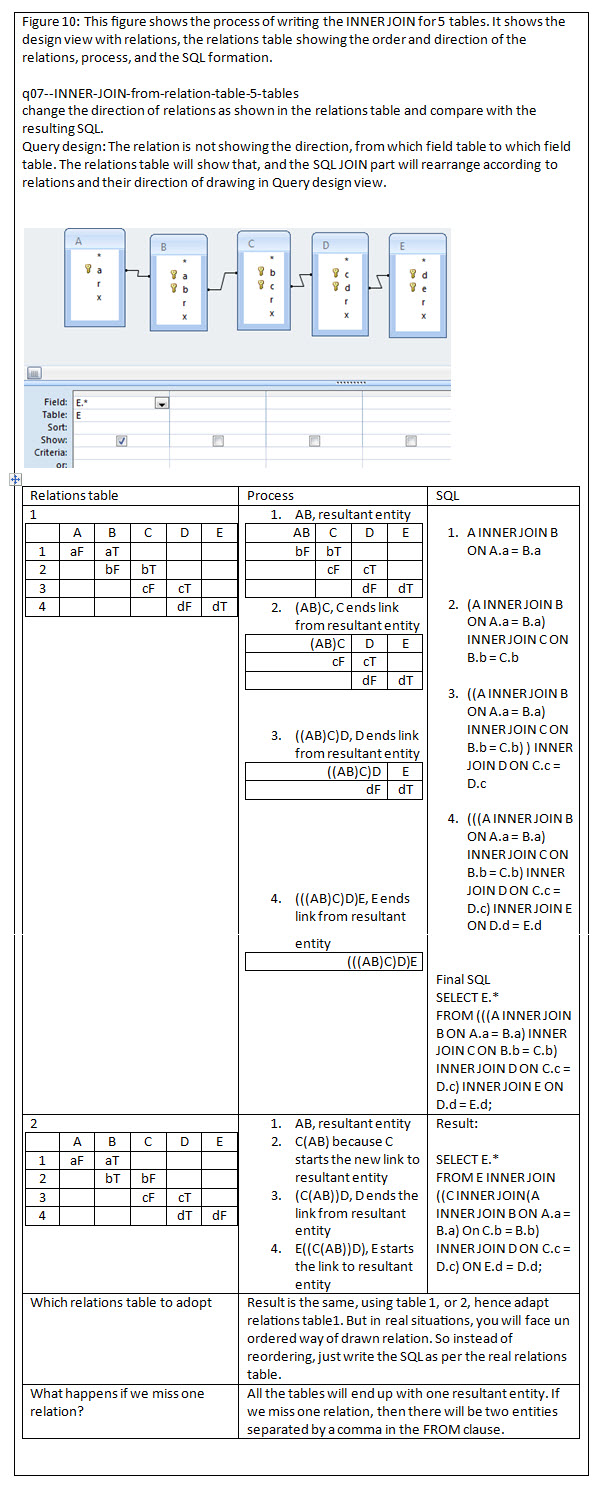Step 10: Writing INNER JOIN SQL from relations table for 4 tables, with last link is completing the join. Figure11.

``````Figure 11: This figure expands on last question happens if we miss one relation? by
adding this relation at end of relations table.

|-----|-----|-----}-----|-----|
|     |  A  |  B  |  C  |  D  |
|-----|-----|-----|-----|-----|
|  1  |  aF | aT  |     |     |
|-----|-----|-----|-----|-----|
|  2  |     |     |  cF | cT  |
|-----|-----|-----|-----|-----|
|  3  |     | bF  |  bT |     |
|-----|-----|-----|-----|-----|

As stated before the 1st and 2nd links will yield two entities (AB), (CD).
The 3rd link INNER Joins the 2 enties producting the following SQL.

SELECT *
FROM (A INNER JOIN B ON A.a = B.a) INNER JOIN (C INNER JOIN D ON C.c = D.c) ON B.b = C.b;
``````

CONCLUSION

We demonstrated a way to write the INNER JOIN part of a SQL statement for few tables. We start from first relation and that gives (from table) INNER JOIN (to table). The next link dictates the position of the 3rd table, if the relation is drawn from it, then it is INNER JOINed to the result of link1, otherwise the result of Link1 is INNER JOINed to it. We repeat the same process with other tables.

If a new  link is added that repeats both from and to sides of a link to tables previously processed,  they are not repeated in the INNER JOIN rather,  we only incorporate the fields in the join conditions.

The results of the joins are the same, if they appear the same in design view, although the arrangement of tables looks different. Following the approach presented simplifies the writing and the debugging of such queries.

If a link is out of order, meaning missing one relation, this will produce a cross join of two entities. And if the last missing link is introduced, the SQL will INNER JOIN the two entities.